# The rationale for fxl and Novel Methods

Single-case research designs involve the presentation of empirical data across experimental conditions to visually draw inferences regarding the effects of environmental manipulations on observed behavior. The empirical data featured in these designs are presented using various conventions that have been shaped by decades of research and practice in behavior analysis. Many of these conventions are highly specialized and are not natively supported in any existing software package (e.g., phase change lines being drawn across multiple individual plots). Even though methods exist for accommodating these conventions in spreadsheet software, these are all tedious and effortful and subsequent modifications typically require additional human interaction to edit. Various scripts and macros have been suggested to streamline these efforts; however, these are at best incomplete at best and a security threat at worst. Indeed, most organizations disable the use of macros by default for all users. The fxl package was designed to meet this gap in available and accessible tools.

## Primary Goals of fxl Package

Various methods exist for visualizing the results of clinical work and research using single-case designs and visual analysis. However, most of these approaches are limited in flexibility, reproducibility, and security. The methods and design of the package have been designed to accomplish several goals.

### Goal #1: Transparency and Replicability

Visualizations of single-case designs are carefully constructed from empirical data to support visual analysis. This is potentially problematic from a reproducibility standpoint, as these types of figures are traditionally constructed by hand and cannot be fully reproduced without direct access to the exact spreadsheet from which the figure was developed. Furthermore, even with direct access to the exact spreadsheet, changes cannot be audited and documented over time and this further hinders efforts at transparency.

The fxl package was designed to address these shortcomings by leveraging the transparency and replicability of the R Statistical Program and supporting the drawing of single case design figures using R syntax only. This syntax-forward approach also allows researchers to pre-register their design specifications and visualizations in an attempt to better distinguish exploratory vs hypothesis testing research.

Manual construction of single case plots, even when using templates, entails an array of monotonous and tedious tasks that must be performed by hand. Various features of these figures must be generated by hand using methods that were not originally designed for such purposes (e.g., combining multiple plots into a single plot). This is not an efficient use of clinical or research time.

The package was developed to provide access to methods specifically designed to feature the conventions not supported elsewhere. These methods are provided ‘out-of-the-box’ to make these conventions more accessible and more efficient for researchers and clinicians.

### Goal #3: Support for Dynamic Reporting

Attempts to scale interventions and clinical/educational programming based on single case designs and visual analysis have traditionally been difficult due to the efforts and consideration required for visual analysis. Generally, most clinicians working with many cases typically track and monitor the results of intervention using individual spreadsheets. That is, much of this work is unnecessarily duplicated and this introduces a significant source of inefficiency at scale.

The fxl package was designed to be compatible with RMarkdown and Quarto to support dynamic reports that can simultaneously visualize progress for multiple individuals (e.g., students in a classroom) as well as multiple groups (e.g., across classrooms in a grade). Specifically, fxl was designed to be able to be used in a way that scaled effectively in ways that other, earlier methods for drawing single case design figures did not. Similarly, this approach can be used to draw up-to-date reports, drawn in lossless quality, and built to scale as new students are added to the caseload.

## Syntax and General Code Structure

The fxl package was constructed to use a functional approach to R and makes heavy use of the `|>` pipe operator to streamline the expressiveness of the syntax. For users of the ggplot2 package, the general logic and workflow are almost identical. That is, a general object is created (e.g., ‘fxl’ class in fxl as opposed to ‘ggproto’ in ggplot2) and layers are added to the object to tailor what is drawn upon the figure (e.g., markers, lines, annotations) and adjust other features of the figure (e.g., spacing, coordinate limits).

### Rationale for Distinct Package/Style

The decision not to simply build upon the well-established ggplot2 package was one that was not made lightly or at haste. Indeed, I tried, and certain conventions in single case design and visual analysis simply are so idiosyncratic that no existing plotting system could accommodate all of them natively. I got about 80% of where I needed to get with ggplot2, but ultimately, I had to create a custom approach to design an approach that would be useful for single case design users.

The high degree of overlap in terms of workflow (i.e., functional style), function calls (e.g., scr_*), and features was deliberate and there were several reasons for doing so. First, the initial design used ggplot2 as a base and focused on extending the package to support conventions common in single-case research designs. However, low-level conventions regarding how individual facets were drawn limited options for drawing cross-plot features (e.g., staggered phase change lines across plots). Considerable work was done here, but a dedicated drawing algorithm using base R methods needed to be developed to fully incorporate all desired plotting features. Simply put, the functionality didn’t seem to exist (without a deep re-write) and the project reached a point where it was easier to make something specific rather than making something designed to be very general (i.e., ggplot2 into something so specialized).

Second, the pragmatic and stylistic features of ggplot2 (to whatever degree helpful) were deliberately maintained to assist users of ggplot2 in using the package. To be clear, this was not done to create an alternative or competitor to ggplot2. If functionality for drawing annotations across multiple plots in a figure (i.e., staggered phase change lines, free drawing of text/shapes/images) were to be added to ggplot2, I’d merge all of this into an extension because that would be easier to manage over time.

### Core Plotting Object

The core plotting object for the figure is produced using the scr_plot call with the required arguments. The required arguments include a valid dataframe with relevant data as well as a list of aesthetics that direct the engine on how to visualize that data (e.g., which data corresponds with the x-axis). Other arguments are also available to further customize the plot (e.g., margins), but only these are required to produce the core object.

#### Required: x

The x argument represents the domain of the data (e.g., sessions, days) and is a required argument. The core object will return an error if this is not set within the aesthetics object (i.e., var_map). The entry is simply the name of the column in the data frame.

#### Required: y

The y argument represents the ordinate of the data (e.g., frequency, rate of behavior) and is a required argument. The core object will return an error if this is not set within the aesthetics object (i.e., var_map). The entry is simply the name of the column in the data frame.

#### Optional: p [Phases]

The p argument represents the phases that correspond with x and y coordinates (e.g., baseline, intervention). This is an optional argument, given that there are multiple ways that different lines/points can be drawn upon plots/figures. Normally, this is most useful for distinguishing between sets of data points that are similar but disconnected in a figure (e.g., different baseline periods in an A-B-A-B reversal design). The entry is simply the name of the column in the data frame.

#### Optional: facet [Participants, Targets, Subplots]

The facet argument represents the individual panels or subplots within the greater figure. That is, these are typically distinguished by varying participants/classrooms (i.e., multiple baseline across subjects) or varying targets/settings (e.g., multiple baseline within an individual). This is critical for visualizing baseline logic but the facet argument is an optional one because not all designs require more than one facet. The entry is simply the name of the column in the data frame.

#### Example Core Plotting Object:

An example of this is provided below:

``````suppressPackageStartupMessages(library(fxl))

scr_plot(
Gilroyetal2015, # Data frame (long format)
aesthetics = var_map(
x = Session, # Column name for x-axis values
y = Responding, # Column name for y-axis values
p = Condition, # Column name for phases associated with x-y coordinates
facet = Participant # Column name distinguishing individual plots/facets
)
)``````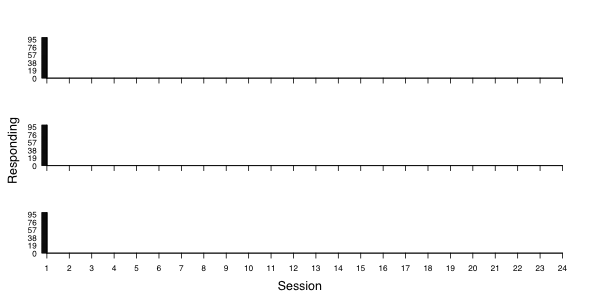### Adding Drawing Layers to Core Plotting Object

The core plotting object alone will rarely provide a sufficient visualization of the supplied data and layers can be added to the object to further refine the end result. That is, despite the core object being provided with x-y coordinate data, no specific instructions to draw are provided without specific instructions. Layers to add the drawing of markers (scr_points), lines (scr_lines), or alter any other relevant feature of the figure must be specified in the call.

Note: The order in which the layers are added are relevant. That is, those layers added earlier in the chain will be drawn earlier and likewise those added later will be drawn later.

#### Data Layers

Across each of the data layers, there is a corresponding x and y argument at minimum. However, there are various options and overrides available. An example that builds from the previous example and adds points and lines is provided below:

``````scr_plot(
Gilroyetal2015,
aesthetics = var_map(
x = Session,
y = Responding,
p = Condition,
facet = Participant
)
) |>
scr_points(cex = 2) |> # plot points, using x/y from aesthetics
scr_lines(size = 1) # plot line, using x/y from aesthetics``````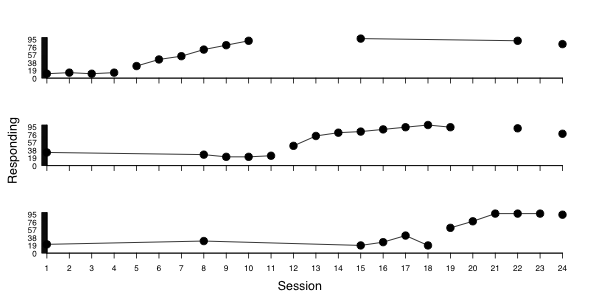As an added note, it warrants stating clearly that the sequence (i.e., the call must begin with scr_plot) and chain of calls are relevant to how the program works (i.e., all are linked together by the native R pipe operator ‘|>’). The pipe operator should be used to connect calls in the sequence; however, the historical ‘%>%’ operator from magittr could also be used if desired (but is not generally recommended). Additionally, the order of layers is also relevant because layers added earlier with be drawn earlier (i.e., lower z-index) and layers added later will be drawn later (i.e., higher z-index).

##### Points: scr_points

At the most basic levels, data can be visualized with markers by using the scr_points layer and add this to the core plotting object. If the basic aesthetics are not overridden (i.e., mapping), this layer will draw the standard x and y references.

##### Cumulative Sum Points: scr_cumsum_points

Related, but distinct from scr_points, the scr_cumsum_points layer will draw points that cumulatively increase in value as data is added to the series (i.e., y data builds upon the prior). If the basic aesthetics are not overridden (i.e., mapping), this layer will draw the standard x and y references.

##### Lines: scr_lines

Often complemented by markers, data can be visualized with markers by using the scr_lines layer and add this to the core plotting object. If the basic aesthetics are not overridden (i.e., mapping), this layer will draw the standard x and y references.

##### Cumulative Sum Lines: scr_cumsum_lines

Related, but distinct from scr_lines, the scr_cumsum_lines layer will draw lines that cumulatively increase in value as data is added to the series (i.e., y data builds upon the prior). If the basic aesthetics are not overridden (i.e., mapping), this layer will draw the standard x and y references.

##### Images: scr_images

As an alternative to built-in markers, data can be visualized with markers by using the scr_images layer and add this to the core plotting object. These images must be valid RGML images, which R needs to draw vector-based content in the plotting area. If the basic aesthetics are not overridden (i.e., mapping), this layer will draw the standard x and y references.

##### Bars: scr_bar_support

The scr_bar_support layer is a support layer. That is, this provides supporting data that complements the primary data. Generally, double y-axis plots are often considered too prone to visual misrepresentation and not formally supported, but this is supported in fxl to allow for the concurrent representation of percentage data along with other forms of data. Specifically, this is most relevant to displays wherein procedural fidelity is critical to the interpretation of the primary x/y data.

#### Annotation Layers

##### Text (within panels): scr_label_phase

The scr_label_phase layer provides a means to draw labels (e.g., “Baseline”, “Intervention”) in a specific plot (i.e., “Participant 1”). For example, it is often customary to provide phase labels within the topmost panel of a multi panel figure as opposed to draw those labels in each panel.

##### Text (across panels): scr_label_facet

The scr_label_facet layer provides a means to draw labels (e.g., “Participant 1”, “Participant 2”) across respective plots in a figure. For example, it is often customary to provide facet labels within each panel of a multi panel figure to indicate which data refers to respective participants/targets/settings.

##### Phase Changes (within panels): scr_plines

Phase change lines are critical to highlighting anticipated contrasts between conditions in single case research designs. The scr_plines layer relates to phase change lines that are within individual participants alone. That is, these are not phase change lines that cut across multiple plots. For example, these may be most common in cases where a dashed phase change line is introduced to highlight a slight alteration to a specific phase (e.g., introduce a small environmental change).

##### Phase Changes (across panels): scr_plines_mbd

The scr_plines_mbd layer relates to phase change lines that are across individual panels. That is, these are phase change lines that cut across multiple plots to illustrate a shared environmental change. These conentions are the traditional means of illustrating experimental control in a multiple panel design and are illustrated with solid, unbroken lines.

##### Arrows: scr_anno_arrows

A common convention in single case designs is to mark up data using arrows and labels in lieu of a formal legend. The scr_anno_arrows layer is used to draw arrows upon the respective (sub)plots convey information to the analyst.

##### Brackets: scr_anno_brackets

Another common convention in single case designs is to mark up runs of data using brackets and labels. For example, this is common in displays that convey progression in various procedures (e.g., demand fading). The scr_anno_brackets layer is used to draw brackets upon the respective (sub)plots convey information to the analyst regarding some progression (e.g., demand fading from 1 to 6).

##### Aim Lines: scr_anno_guide_line

Various single case designs in education mark up future (or projected) runs of data using aim lines. For example, this is common in displays that communicate a desired response to educational intervention (e.g., reading fluency expected at the end of the year). The scr_anno_guide_line layer is used to draw lines upon the respective (sub)plots convey information to the analyst regarding some idealized rate of change from a certain point in time to another (e.g., beginning of intervention to end of school year).

##### Legend: scr_legend

The traditional means of orienting the analyst to data in single case research designs rely on legends to communicate what markers and lines corresponded with on a figure. The scr_legend layer is used to draw bespoke legends to communicate respective information on the figure.

#### Plotting Overrides

##### Title: scr_title

The scr_title overrides the default figure title with custom formatting, text, and aesthetics.

##### Label: scr_xlabel

The scr_xlabel overrides the default, common x axis with custom formatting, text, and aesthetics.

##### Label: scr_ylabel

The scr_ylabel overrides the default, common y axis with custom formatting, text, and aesthetics.

##### Ticks: scr_xoverride

The scr_xoverride overrides the tick marks and tick labels for (sub)plots in a greater figure.

##### Ticks: scr_yoverride

The scr_yoverride overrides the tick marks and tick labels for (sub)plots in a greater figure.

#### General Layer Aesthetics

##### Color (color)

The colors of text, lines, and markers can be overridden (typically ‘black’ by default) by specifying the color in the layer call.

##### Line Type (lty)

The line type (typically solid or ‘1’) can be overridden by specifying the lty in the layer call.

##### Line Width (size)

The line width (typically 1) can be overridden by specifying the size in the layer call.

##### Aesthetics (mapping)

Generally, the primary aesthetics (e.g., x, y, p, facet) are carried forward into specific layers (e.g., points, lines). The aesthetics for a specific layer can be overridden by specifying a particular mapping for a respective layer.

An example of this is provided below:

``````scr_plot(
Gilroyetal2015,
aesthetics = var_map(
x = Session,
y = Responding,
p = Condition,
facet = Participant
)
) |>
scr_points(cex = 2) |>
scr_lines(size = 1) |>
scr_xoverride(c(0.25, 27.5),
xticks = 1:27,
xtickslabs = as.character(1:27)
) |> # manually override x-axis (make extra room for labels) and specify ticks
scr_yoverride(c(-5, 105),
yticks = c(0, 50, 100),
ytickslabs = as.character(c(0, 50, 100)),
) |> # manually override y-axis and tick interval (tick for every 10 units)
scr_xlabel("Session") |> # Override x-axis label (bottom only shown by default)
scr_ylabel("Percent Accuracy") |> # Override y-axis label (centered, leftmost label)
scr_title("Rates of Acquisition across Participants")``````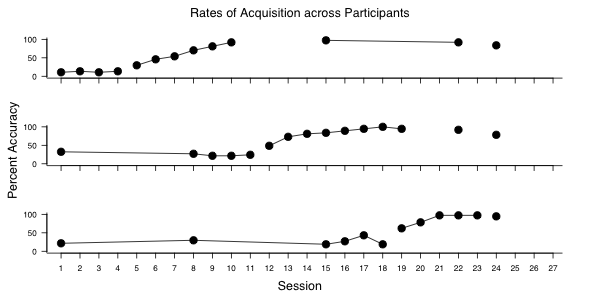# Publication Quality Examples using fxl

Several examples using fxl and data from peer-reviewed works are provided in the sections below. The source code necessary to reproduce all figures here is provided in the ‘demo’ folder of the source repository.

## Gilroy et al. (2021) - Operant Behavioral Economics

This example uses a concurrent reversal design to systematically evaluating how different types of reinforcement schedules derived from demand assessments influence learner performance.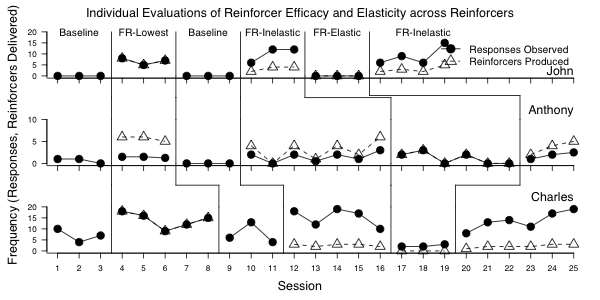## Gilroy et al. (2019) - Functional Communication Training

This example features a functional analysis figure as well as a hybrid design that combines a multiple baseline approach and a reversal to establish multiple instances of experimental control.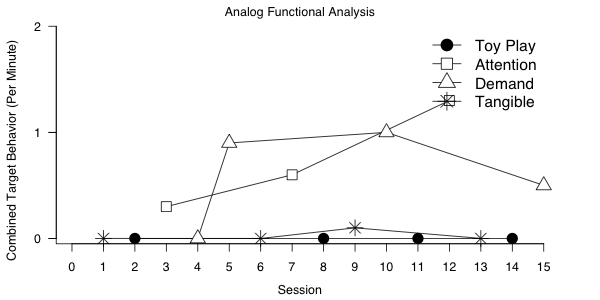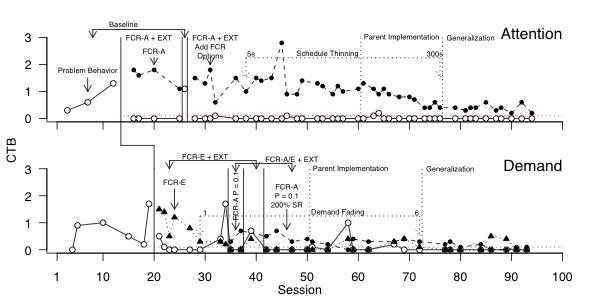## Gilroy et al. (2015) - Derived Relational Responding

This example uses a multiple baseline design (multiple probe, specifically) to establish multiple instances of experimental control.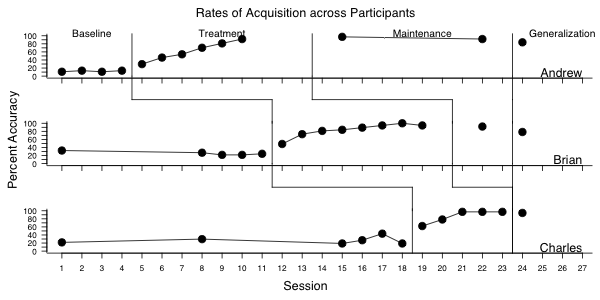# Automation of fxl in Clinical Reports

Apart from research applications and the benefits afforded by a purely programmatic means of building, generating, and archiving figures, these benefits also extend to various types of clinical applications as well. That is, any type of regularly collected data and form of educational programming that is common across students or cases can be visualized in a way that (1) reduces redundancy and eliminates the need for individual spreadsheets, (2) can be updated moment-to-moment with either the click of a button or as an automated process (e.g., run every morning before classes start), or (3) facilitates an overall view of the individual’s behavior on its own and in comparison to the rest of the class/caseload.

The ability to generate up-to-date reports and present data ready for visual analysis assists analysts in using data to inform their decisions as well as to learn more about present needs. Reports can be used to facilitate high-level reviews of behavior change (e.g., across center, school, grade level) as well as inspection of individual-level response to intervention and progress monitoring.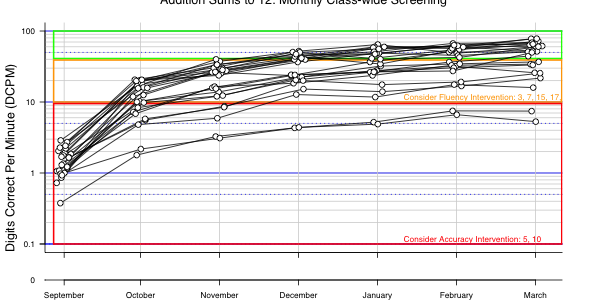### Individualized Inspection of Student Learning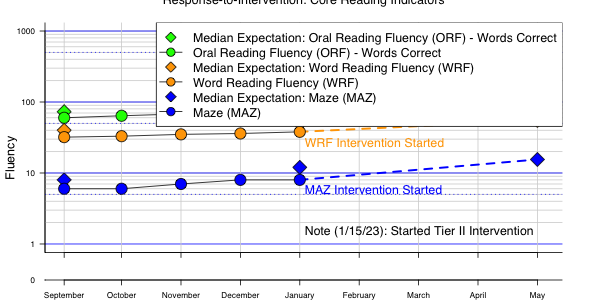# Descriptive Use Cases

Descriptive use cases are provided here to provide ‘from the ground up’ demonstrations of how to use fxl to generate visual figures. A range of use cases will be provided to demonstrate the research and clinical applications of the package.

## Use Case Number 1: Basic Treatment Evaluation with Reversal Design

The most fundamental and applicable design is the reversal design, which has strong representation in both clinical and applied contexts. In clinical contexts, this is often the quickest means of evaluating whether a particular treatment has the desired effect on specific behavior.

To be consistent with behavior analytic conventions this type of figure would involve featuring:

• One or more data layers with markers and lines

• Phase change lines

• Phase labels

• Informative axis labels

• Series annotations and/or a figure legend

A short tutorial regarding the design and execution of each convention is provided in the section below.

### Data Import

The data relevant to the figure must be imported in R in the form of a data frame prior to generating a plotting object. By default, fxl works with wide data because this is generally how most folks trained to work within spreadsheets arrange and organize their data (i.e., individual data series have their own columns).

An example of this data formatting is provided below:

``````use_case_1 <- data.frame(
Session = seq_len(16),
Target = c(
runif(3, 10, 20),
runif(3, 0, 10),
runif(3, 10, 20),
runif(7, 2, 8)
),
Phase = c(
rep("Baseline", 3),
rep("Intervention", 3),
rep("Baseline2", 3),
rep("Intervention2", 7)
),
Participant = rep("1", 16)
)

``````##   Session   Target    Phase Participant
## 1       1 16.47243 Baseline           1
## 2       2 13.93434 Baseline           1
## 3       3 16.66269 Baseline           1``````

In this example, there is a column specific to the x-axis (Session) and the y-axis (Target) as well as a variable to speaks to data embedded within a specific condition (Phase). The Phase factor must have unique tags for each phase (i.e., “baseline” vs “baseline2”), otherwise it would try to connect points between two phases that were not temporally connected (i.e., connecting the last point of the first baseline to the first point of the second baseline).

### Mapping Aesthetics to Data

The current data can be mapped using the var_map function to assign the x-axis variable (Session), the y-axis variable (at least a primary one; Target), and the phase identifier (Phase). The result of this, without any specific data layers, is presented below.

``````scr_plot(
use_case_1,
aesthetics = var_map(
x = Session,
y = Target,
p = Phase
)
)``````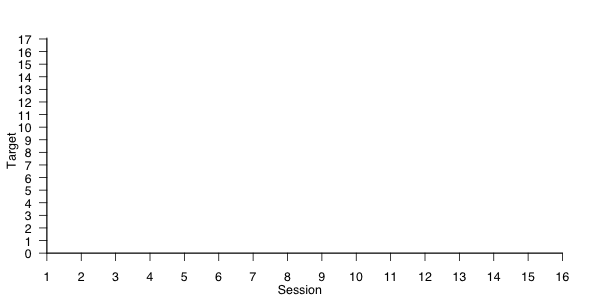By default, assigning the core plot alone will only create the plotting space with default labels. A simple, single series of lines and markers can be added to the core plotting object by adding the scr_points and scr_lines layers.

``````scr_plot(use_case_1,
aesthetics = var_map(
x = Session,
y = Target,
p = Phase
)
) |>
scr_lines() |>
scr_points()``````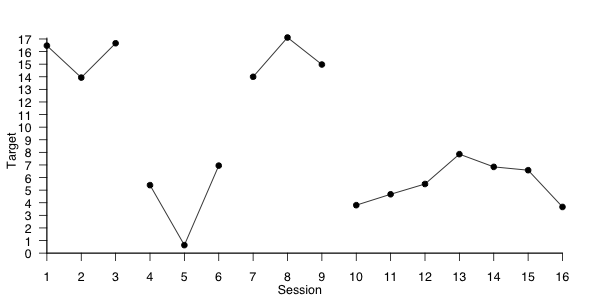### Customizing Margins and Axes

The plotting engine will be intelligent ‘guesses’ as to margin sizes, but these will often have to be overridden to make the overall display more pleasing. That is, the ticks on the y-axis may need to be adjusted to become less cluttered as well (i.e., larger interval between ticks) and padding may be helpful to provide separation from the origin (i.e., 0-point). An example of this provided in the space below.

``````scr_plot(use_case_1,
aesthetics = var_map(
x = Session,
y = Target,
p = Phase
),
mai = c(
0.375,
0.5,
0.175,
0.1
),
omi = c(
0.25,
0.25,
0.25,
0.25
)
) |>
scr_xoverride(
c(0.5, 16.5),
xticks = 1:16
) |>
scr_yoverride(
c(-0.5, 20),
yticks = c(0, 5, 10, 15, 20),
ydelta = 5
) |>
scr_lines() |>
scr_points()``````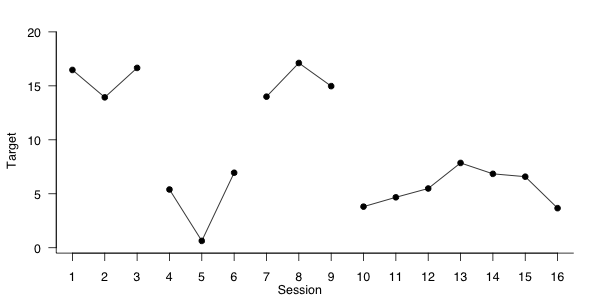Adding phase changes lines to the current can be done in one of two ways: adding to individual facets (e.g., just in one subplot; scr_plines) or as an element that cuts across multiple facets (e.g., across three plots in a figure; scr_plines_mbd). Since the current example has only one facet, the scr_plines layer is the most appropriate.

The current figure involves three elements, separating the four phases, and each element is described using a specific key-ed value in the call. Note: since we extended out the bottom of the y-axis, we must add an argument (y2 = -0.5) to make sure this matches the current floor for the figure in each of the phase change elements.

``````scr_plot(use_case_1,
aesthetics = var_map(
x = Session,
y = Target,
p = Phase
),
mai = c(
0.375,
0.5,
0.175,
0.1
),
omi = c(
0.25,
0.25,
0.25,
0.25
)
) |>
scr_xoverride(
c(0.5, 16.5),
xticks = 1:16
) |>
scr_yoverride(
c(-0.5, 20),
yticks = c(0, 5, 10, 15, 20),
ydelta = 5
) |>
scr_lines() |>
scr_points() |>
scr_plines(
lty = 1,
lines = list(
"A" = list(
x1 = 3.5,
y1 = 20,
y2 = -0.5
),
"B" = list(
x1 = 6.5,
y1 = 20,
y2 = -0.5
),
"C" = list(
x1 = 9.5,
y1 = 20,
y2 = -0.5
)
)
)``````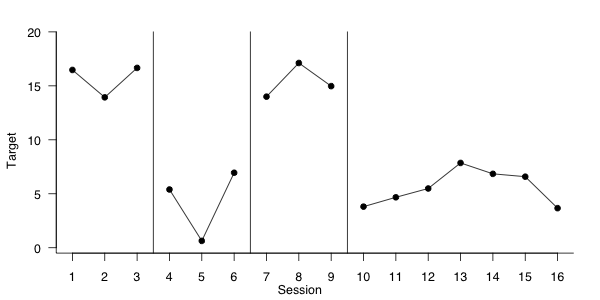The figure can be made more descriptive by drawing text in certain areas to designate which phases correspond with certain changes in the environment. Adding labels to the figure can be done by drawing them within a single plot (i.e., scr_label_phase) or individually across multiple facets (i.e., scr_label_facet). Since there is only one subplot in this figure, the scr_label_phase call is more appropriate.

The current figure involves four phases, two instances of baseline and intervention, respectively. Labels are drawn using a series of key-ed list elements, with each element corresponding to a unique label. Note: since we have repeated instances of similar conditions, we must override the label argument in the key-ed entry within each of the items.

``````scr_plot(use_case_1,
aesthetics = var_map(
x = Session,
y = Target,
p = Phase
),
mai = c(
0.375,
0.5,
0.175,
0.1
),
omi = c(
0.25,
0.25,
0.25,
0.25
)
) |>
scr_xoverride(
c(0.5, 16.5),
xticks = 1:16
) |>
scr_yoverride(
c(-0.5, 20),
yticks = c(0, 5, 10, 15, 20),
ydelta = 5
) |>
scr_lines() |>
scr_points() |>
scr_plines(
lty = 1,
lines = list(
"A" = list(
x1 = 3.5,
y1 = 20,
y2 = -0.5
),
"B" = list(
x1 = 6.5,
y1 = 20,
y2 = -0.5
),
"C" = list(
x1 = 9.5,
y1 = 20,
y2 = -0.5
)
)
) |>
scr_label_phase(
cex = 1,
face = 2,
y = 20,
labels = list(
"A" = list(
x = 2,
label = "Baseline"
),
"B" = list(
x = 5,
label = "Intervention"
),
"C" = list(
x = 8,
label = "Baseline"
),
"D" = list(
x = 13,
label = "Intervention"
)
)
)``````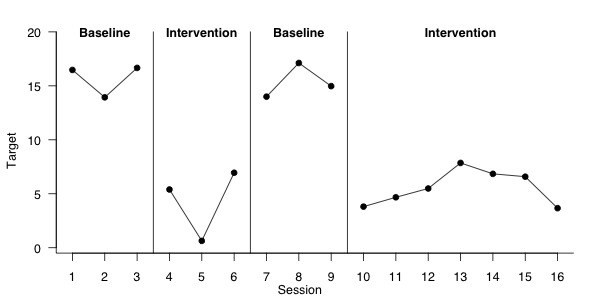### Customizing Title, Axes Labels

As a final point of styling this figure, the specific labels for each axis can be customized to better suit the desired theme. Generally, there are three primary features that might need adjustment: scr_xlabel, scr_ylabel, and scr_title. Each of these corresponds to the respective label.

An example customizing these features is provided in the section below.

``````scr_plot(use_case_1,
aesthetics = var_map(
x = Session,
y = Target,
p = Phase
),
mai = c(
0.375,
0.5,
0.175,
0.1
),
omi = c(
0.25,
0.25,
0.25,
0.25
)
) |>
scr_xoverride(
c(0.5, 16.5),
xticks = 1:16
) |>
scr_yoverride(
c(-0.5, 20),
yticks = c(0, 5, 10, 15, 20),
ydelta = 5
) |>
scr_lines() |>
scr_points() |>
scr_plines(
lty = 1,
lines = list(
"A" = list(
x1 = 3.5,
y1 = 20,
y2 = -0.5
),
"B" = list(
x1 = 6.5,
y1 = 20,
y2 = -0.5
),
"C" = list(
x1 = 9.5,
y1 = 20,
y2 = -0.5
)
)
) |>
scr_label_phase(
y = 20,
labels = list(
"A" = list(
x = 2,
label = "Baseline"
),
"B" = list(
x = 5,
label = "Intervention"
),
"C" = list(
x = 8,
label = "Baseline"
),
"D" = list(
x = 13,
label = "Intervention"
)
)
) |>
scr_xlabel("Daily Sessions") |>
scr_ylabel("Rates of Target Behavior (per Minute)") |>
scr_title("Use Case #1",
face = 2
)``````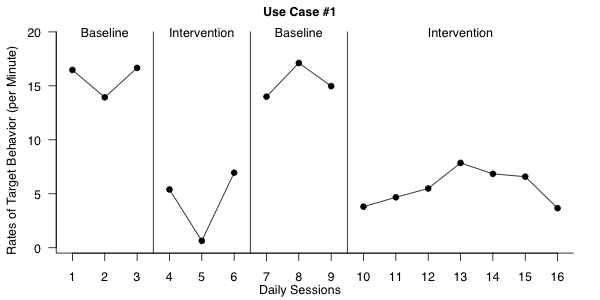### Saving Figure Output

After the desired design is complete, the figure drawn within R can be saved in any number of formats (e.g., rasters, vectors) depending on the need (e.g., insert into an educational report, saved to include in manuscript). It is important to think carefully about the dimensions supplied for the output. That is, smaller figures will present as “cramped” and may not provide sufficient space in the plane to include suitable text sizes.

An example that outputs the current figure to a PNG-type file is provided in the section below.

``````scr_plot(use_case_1,
aesthetics = var_map(
x = Session,
y = Target,
p = Phase
),
mai = c(
0.375,
0.5,
0.175,
0.1
),
omi = c(
0.25,
0.25,
0.25,
0.25
)
) |>
scr_xoverride(
c(0.5, 16.5),
xticks = 1:16
) |>
scr_yoverride(
c(-0.5, 20),
yticks = c(0, 5, 10, 15, 20),
ydelta = 5
) |>
scr_lines() |>
scr_points() |>
scr_plines(
lty = 1,
lines = list(
"A" = list(
x1 = 3.5,
y1 = 20,
y2 = -0.5
),
"B" = list(
x1 = 6.5,
y1 = 20,
y2 = -0.5
),
"C" = list(
x1 = 9.5,
y1 = 20,
y2 = -0.5
)
)
) |>
scr_label_phase(
y = 20,
labels = list(
"A" = list(
x = 2,
label = "Baseline"
),
"B" = list(
x = 5,
label = "Intervention"
),
"C" = list(
x = 8,
label = "Baseline"
),
"D" = list(
x = 13,
label = "Intervention"
)
)
) |>
scr_xlabel("Daily Sessions") |>
scr_ylabel("Rates of Target Behavior (per Minute)") |>
scr_title("Use Case #1",
face = 2
) # |>````````````#   scr_save(
#     name = "use_case_1.png",
#     units = "in",
#     width = 9,
#     height = 6,
#     format = "png"
#   )``````

### Closing and Summary

This use case serves as a good introduction to the fxl package in that the reversal design is very common across both research and applied settings. Using this example as a base, one could easily adapt this snippet and expand its elements to fits various clinical, educational, and/or research needs.

## Use Case Number 2: Multiple Baseline Design across Students

The multiple baseline design is among the most common research designs used in educational settings. This particular design has good utility in both research and clinical applications. However, the use of multiple concurrent (or nonconcurrent) data series presents an issue wherein most graphical charting packages do not support cross-plot conventions natively. Historically, most analysts have had to rely on over-engineered spreadsheets and highly specialized customizations to produce the desired figure.

To be consistent with behavior analytic conventions this type of figure would involve featuring:

• One or more data layers with markers and lines

• Phase change lines

• Phase labels

• Informative axis labels

• Series annotations and/or a figure legend

• Phase change lines that distinguish conditions within as well as between individual subplots.

A short tutorial regarding the design and execution of each convention is provided in the section below.

### Data Import

The data relevant to the figure must be imported in R in the form of a data frame prior to generating a plotting object. By default, fxl works with wide data because this is generally how most folks trained to work within spreadsheets arrange and organize their data (i.e., individual data series have their own columns).

An example of this data formatting is provided below:

``````use_case_2 <- Gilroyetal2015

``````##   Participant Session Condition Responding PhaseNum LineOff
## 1      Andrew       1  Baseline   10.81081        1       0
## 2      Andrew       2  Baseline   13.51351        1       0
## 3      Andrew       3  Baseline   10.81081        1       0``````

In this example, there is a column specific to the x-axis (Session) and the y-axis (Responding) as well as a variable to speaks to data embedded within a specific condition (Condition). The Phase factor must have unique tags for each phase (i.e., “1” vs “2”), otherwise it would try to connect points between two phases that were not temporally connected (i.e., connecting the last point of the first baseline to the first point of the second baseline).

### Mapping Aesthetics to Data

The current data can be mapped using the var_map function to assign the x-axis variable (Session), the y-axis variable (at least a primary one; Responding), and the phase identifier (Condition). The result of this, without any specific data layers, is presented below.

``````scr_plot(
use_case_2,
aesthetics = var_map(
x = Session,
y = Responding,
p = Condition,
facet = Participant
),
mai = c(
0.375,
0.375,
0.2,
0.0
),
omi = c(
0.25,
0.25,
0.25,
0.1
)
) |>
scr_xoverride(
c(0.25, 27.5),
xticks = 1:27,
xtickslabs = as.character(1:27)
) |> # manually override x-axis (make extra room for labels)
scr_yoverride(
c(-5, 105), # manually override y-axis and tick interval (tick every 10 units)
yticks = seq(0, 100, by = 10),
ytickslabs = as.character(seq(0, 100, by = 10)),
)``````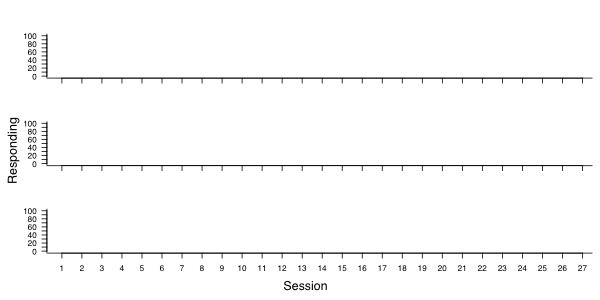By default, assigning the core plot alone will only create the plotting space with default labels. A simple, single series of lines and markers can be added to the core plotting object by adding the scr_points and scr_lines layers.

``````scr_plot(
use_case_2,
aesthetics = var_map(
x = Session,
y = Responding,
p = Condition,
facet = Participant
),
mai = c(
0.375,
0.375,
0.2,
0.0
),
omi = c(
0.25,
0.25,
0.25,
0.1
)
) |>
scr_xoverride(
c(0.25, 27.5),
xticks = 1:27,
xtickslabs = as.character(1:27)
) |> # manually override x-axis (make extra room for labels)
scr_yoverride(
c(-5, 105), # manually override y-axis and tick interval (tick every 10 units)
yticks = seq(0, 100, by = 10),
ytickslabs = as.character(seq(0, 100, by = 10)),
) |>
scr_lines() |>
scr_points(cex = 2)``````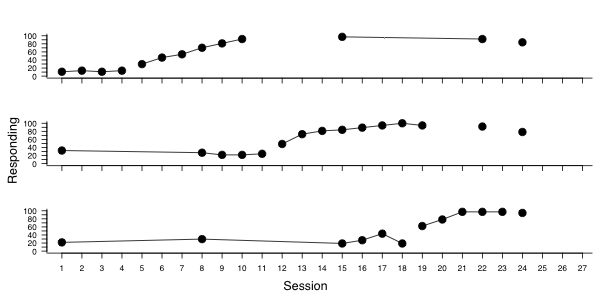Adding phase changes lines to the current can be done in one of two ways: adding to individual facets (e.g., just in one subplot; scr_plines) or as an element that cuts across multiple facets (e.g., across three plots in a figure; scr_plines_mbd). Since the current example has three facets, illustrating a staggered introduction of some independent variable, the scr_plines_mbd layer is the most appropriate.

The current figure involves three elements, separating the phases across the three panels, and each cross-figure phase change point is described using a key-ed value in the call. Specifically there are three phase change elements representing the (“A”) boundary between baseline and intervention, (“B”) intervention and maintenance, and (“C”) maintenance and generalization. The specific keys for the lines are arbitrary; however, the lists must contain named lists that use the facet as the name (e.g., “Andrew”, “Brian”, “Charles”) and include relevant x and y information. Note: since we extended out the bottom of the y-axis, we must add an argument (y2 = -5) to the list for Charles to make sure this matches the current floor for the figure in each of the phase change elements–this generally only matters for the final plot as the connection to phases below this point generally accomplishes this without intervention.

``````scr_plot(
use_case_2,
aesthetics = var_map(
x = Session,
y = Responding,
p = Condition,
facet = Participant
),
mai = c(
0.375,
0.375,
0.2,
0.0
),
omi = c(
0.25,
0.25,
0.25,
0.1
)
) |>
scr_xoverride(
c(0.25, 27.5),
xticks = 1:27,
xtickslabs = as.character(1:27)
) |> # manually override x-axis (make extra room for labels)
scr_yoverride(
c(-5, 105), # manually override y-axis and tick interval (tick every 10 units)
yticks = seq(0, 100, by = 10),
ytickslabs = as.character(seq(0, 100, by = 10)),
) |>
scr_lines() |>
scr_points(cex = 2) |>
scr_plines_mbd(
lines = list( # plot linked phase lines (note: drawn from top through bottom)
"A" = list(
"Andrew" = list(
x1 = 4.5,
y1 = 100
),
"Brian" = list(
x1 = 11.5,
y1 = 100
),
"Charles" = list(
x1 = 18.5,
y1 = 100,
y2 = -5
)
),
"B" = list(
"Andrew" = list(
x1 = 13.5,
y1 = 100
),
"Brian" = list(
x1 = 20.5,
y1 = 100
),
"Charles" = list(
x1 = 23.5,
y1 = 100,
y2 = -5
)
),
"C" = list(
"Andrew" = list(
x1 = 23.5,
y1 = 100
),
"Brian" = list(
x1 = 23.5,
y1 = 100
),
"Charles" = list(
x1 = 23.5,
y1 = 100,
y2 = -5
)
)
)
)``````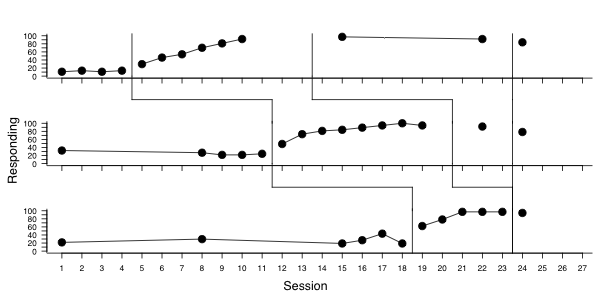### Adding Facet and Phase Labels

The figure can be made more descriptive by drawing text in certain areas to designate which phases correspond with certain changes in the environment and which facets (i.e., sub-plots) correspond with specific participants/targets. Adding labels to the figure can be done by drawing them within a single plot (i.e., scr_label_phase) or individually across multiple facets (i.e., scr_label_facet). Since there are three subplots in this figure, the scr_label_phase and scr_label_facet calls are more necessary.

The current figure features three participants (i.e., “Andrew”, “Brian”, “Charles”) and the plots are illustrated in that order. The scr_label_facet is called with a labels argument and the named lists must correspond with the values for the subplot (e.g., “Andrew”). Each of these arguments must feature information regarding where to draw that label. Note: it is possible to set a general x or y argument in the overall layer as well as to override the label argument in the named list (i.e., if differing from the name for the list/facet).

The figure features four phases across each of the facets–baseline, intervention, maintenance, and generalization. Phase labels are drawn using a series of key-ed list elements, with each element corresponding to a unique label drawn somewhere on the figure. However, since this plot involves multiple subplots in the overall figure, the analyst must specify which facet to draw these labels upon. That is, the facet argument in scr_labels_phase must be set to “Andrew” to specify that phase labels will be drawn on the topmost facet only.

``````scr_plot(
use_case_2,
aesthetics = var_map(
x = Session,
y = Responding,
p = Condition,
facet = Participant
),
mai = c(
0.375,
0.375,
0.2,
0.0
),
omi = c(
0.25,
0.25,
0.25,
0.1
)
) |>
scr_xoverride(
c(0.25, 27.5),
xticks = 1:27,
xtickslabs = as.character(1:27)
) |> # manually override x-axis (make extra room for labels)
scr_yoverride(
c(-5, 105), # manually override y-axis and tick interval (tick every 10 units)
yticks = seq(0, 100, by = 10),
ytickslabs = as.character(seq(0, 100, by = 10)),
) |>
scr_lines() |>
scr_points(cex = 2) |>
scr_plines_mbd(
lines = list( # plot linked phase lines (note: drawn from top through bottom)
"A" = list(
"Andrew" = list(
x1 = 4.5,
y1 = 100
),
"Brian" = list(
x1 = 11.5,
y1 = 100
),
"Charles" = list(
x1 = 18.5,
y1 = 100,
y2 = -5
)
),
"B" = list(
"Andrew" = list(
x1 = 13.5,
y1 = 100
),
"Brian" = list(
x1 = 20.5,
y1 = 100
),
"Charles" = list(
x1 = 23.5,
y1 = 100,
y2 = -5
)
),
"C" = list(
"Andrew" = list(
x1 = 23.5,
y1 = 100
),
"Brian" = list(
x1 = 23.5,
y1 = 100
),
"Charles" = list(
x1 = 23.5,
y1 = 100,
y2 = -5
)
)
)
) |>
scr_label_facet(
cex = 1.5, # plot labels across facets (not within a single facet)
y = 10,
labels = list( # list of labels to draw (will use assigned key for label)
"Andrew" = list(
x = 27
),
"Brian" = list(
x = 27
),
"Charles" = list(
x = 27
)
)
) |>
scr_label_phase(
facet = "Andrew", # plot labels on specific facet
cex = 1.25,
y = 107,
labels = list( # list of labels to draw (will use assigned key for label)
"Baseline" = list(
x = 2.5
),
"Treatment" = list(
x = 9
),
"Maintenance" = list(
x = 19
),
"Generalization" = list(
x = 26
)
)
)``````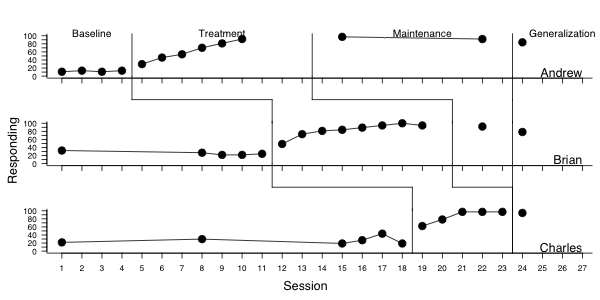### Customizing Title, Axes Labels

As a final point of styling this figure, the specific labels for each axis can be customized to better suit the desired theme. Generally, there are three primary features that might need adjustment: scr_xlabel, scr_ylabel, and scr_title. Each of these corresponds to the respective label.

An example customizing these features is provided in the section below.

``````scr_plot(
use_case_2,
aesthetics = var_map(
x = Session,
y = Responding,
p = Condition,
facet = Participant
),
mai = c(
0.375,
0.375,
0.2,
0.0
),
omi = c(
0.25,
0.25,
0.25,
0.1
)
) |>
scr_xoverride(
c(0.25, 27.5),
xticks = 1:27,
xtickslabs = as.character(1:27)
) |> # manually override x-axis (make extra room for labels)
scr_yoverride(
c(-5, 105), # manually override y-axis and tick interval (tick every 10 units)
yticks = seq(0, 100, by = 10),
ytickslabs = as.character(seq(0, 100, by = 10)),
) |>
scr_lines() |>
scr_points(cex = 2) |>
scr_plines_mbd(
lines = list( # plot linked phase lines (note: drawn from top through bottom)
"A" = list(
"Andrew" = list(
x1 = 4.5,
y1 = 100
),
"Brian" = list(
x1 = 11.5,
y1 = 100
),
"Charles" = list(
x1 = 18.5,
y1 = 100,
y2 = -5
)
),
"B" = list(
"Andrew" = list(
x1 = 13.5,
y1 = 100
),
"Brian" = list(
x1 = 20.5,
y1 = 100
),
"Charles" = list(
x1 = 23.5,
y1 = 100,
y2 = -5
)
),
"C" = list(
"Andrew" = list(
x1 = 23.5,
y1 = 100
),
"Brian" = list(
x1 = 23.5,
y1 = 100
),
"Charles" = list(
x1 = 23.5,
y1 = 100,
y2 = -5
)
)
)
) |> # plot lines, using x/y from aesthetics
scr_label_phase(
facet = "Andrew", # plot labels on specific facet
cex = 1.25,
y = 107,
labels = list( # list of labels to draw (will use assigned key for label)
"Baseline" = list(
x = 2.5
),
"Treatment" = list(
x = 9
),
"Maintenance" = list(
x = 19
),
"Generalization" = list(
x = 26
)
)
) |>
scr_xlabel("Session") |> # Override x-axis label (bottom only shown by default)
scr_ylabel("      Percent Accuracy") |> # Override y-axis label (centered, leftmost label)
scr_title("Rates of Acquisition across Participants",
face = 2
)``````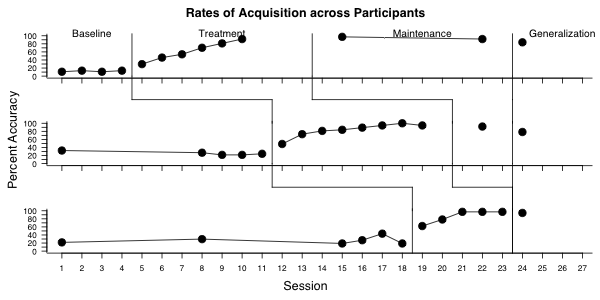### Saving Figure Output

After the desired design is complete, the figure drawn within R can be saved in any number of formats (e.g., rasters, vectors) depending on the need (e.g., insert into an educational report, saved to include in manuscript). It is important to think carefully about the dimensions supplied for the output. That is, smaller figures will present as “cramped” and may not provide sufficient space in the plane to include suitable text sizes.

An example that outputs the current figure to a PNG-type file is provided in the section below.

``````scr_plot(
use_case_2,
aesthetics = var_map(
x = Session,
y = Responding,
p = Condition,
facet = Participant
),
mai = c(
0.375,
0.375,
0.2,
0.0
),
omi = c(
0.25,
0.25,
0.25,
0.1
)
) |>
scr_xoverride(
c(0.25, 27.5),
xticks = 1:27,
xtickslabs = as.character(1:27)
) |> # manually override x-axis (make extra room for labels)
scr_yoverride(
c(-5, 105), # manually override y-axis and tick interval (tick every 10 units)
yticks = seq(0, 100, by = 10),
ytickslabs = as.character(seq(0, 100, by = 10)),
) |>
scr_lines() |>
scr_points(cex = 2) |>
scr_plines_mbd(
lines = list( # plot linked phase lines (note: drawn from top through bottom)
"A" = list(
"Andrew" = list(
x1 = 4.5,
y1 = 100
),
"Brian" = list(
x1 = 11.5,
y1 = 100
),
"Charles" = list(
x1 = 18.5,
y1 = 100,
y2 = -5
)
),
"B" = list(
"Andrew" = list(
x1 = 13.5,
y1 = 100
),
"Brian" = list(
x1 = 20.5,
y1 = 100
),
"Charles" = list(
x1 = 23.5,
y1 = 100,
y2 = -5
)
),
"C" = list(
"Andrew" = list(
x1 = 23.5,
y1 = 100
),
"Brian" = list(
x1 = 23.5,
y1 = 100
),
"Charles" = list(
x1 = 23.5,
y1 = 100,
y2 = -5
)
)
)
) |> # plot lines, using x/y from aesthetics
scr_label_phase(
facet = "Andrew", # plot labels on specific facet
cex = 1.25,
y = 107,
labels = list( # list of labels to draw (will use assigned key for label)
"Baseline" = list(
x = 2.5
),
"Treatment" = list(
x = 9
),
"Maintenance" = list(
x = 19
),
"Generalization" = list(
x = 26
)
)
) |>
scr_xlabel("Session") |> # Override x-axis label (bottom only shown by default)
scr_ylabel("      Percent Accuracy") |> # Override y-axis label (centered, leftmost label)
scr_title("Rates of Acquisition across Participants",
face = 2
) # |>``````